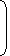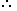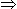# Tips and Tricks to solve Simple Interest Problems1. Principal:The money borrowed or lent out for a certain period is called the principal or the sum.
2. Interest:Extra money paid for using other’s money is called interest.
3. Simple Interest (S.I.):If the interest on a sum borrowed for certain period is reckoned uniformly, then it is called simple interest.Let Principal = P, Rate = R% per annum (p.a.) and Time = T years. Then
 (i). Simple Intereest =P x R x T100
 (ii). P =100 x S.I.; R =100 x S.I.and T =100 x S.I.. R x T P x T P x R

Simple Interest – General Question

1. A sum of money at simple interest amounts to Rs. 815 in 3 years and to Rs. 854 in 4 years. The sum is:
 A. Rs. 650 B. Rs. 690 C. Rs. 698 D. Rs. 700

Answer: Option C

Explanation:

S.I. for 1 year = Rs. (854 – 815) = Rs. 39.

S.I. for 3 years = Rs.(39 x 3) = Rs. 117.Principal = Rs. (815 – 117) = Rs. 698.

2. Mr. Thomas invested an amount of Rs. 13,900 divided in two different schemes A and B at the simple interest rate of 14% p.a. and 11% p.a. respectively. If the total amount of simple interest earned in 2 years be Rs. 3508, what was the amount invested in Scheme B?
 A. Rs. 6400 B. Rs. 6500 C. Rs. 7200 D. Rs. 7500 E. None of these

Answer: Option A

Explanation:

Let the sum invested in Scheme A be Rs. x and that in Scheme B be Rs. (13900 – x).

 Then,x x 14 x 2+(13900 – x) x 11 x 2= 3508 100 10028x – 22x = 350800 – (13900 x 22)6x = 45000x = 7500.

So, sum invested in Scheme B = Rs. (13900 – 7500) = Rs. 6400.

2. Mr. Thomas invested an amount of Rs. 13,900 divided in two different schemes A and B at the simple interest rate of 14% p.a. and 11% p.a. respectively. If the total amount of simple interest earned in 2 years be Rs. 3508, what was the amount invested in Scheme B?
 A. Rs. 6400 B. Rs. 6500 C. Rs. 7200 D. Rs. 7500 E. None of these

Answer: Option A

Explanation:

Let the sum invested in Scheme A be Rs. x and that in Scheme B be Rs. (13900 – x).

 Then,x x 14 x 2+(13900 – x) x 11 x 2= 3508 100 10028x – 22x = 350800 – (13900 x 22)6x = 45000x = 7500.

So, sum invested in Scheme B = Rs. (13900 – 7500) = Rs. 6400.

Correct! Wrong!

Correct! Wrong!

Correct! Wrong!

Correct! Wrong!

#### An automobile financier claims to be lending money at simple interest, but he includes the interest every six months for calculating the principal. If he is charging an interest of 10%, the effective rate of interest becomes

Correct! Wrong!

QUIZ ON SIMPLE INTEREST
EXCELLENT!!!

Share your Results:

Don't miss out!
Subscribe To Our Newsletter

Learn new things. Get an article everyday.

Invalid email address
Give it a try. You can unsubscribe at any time.